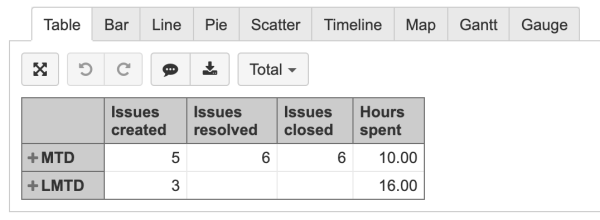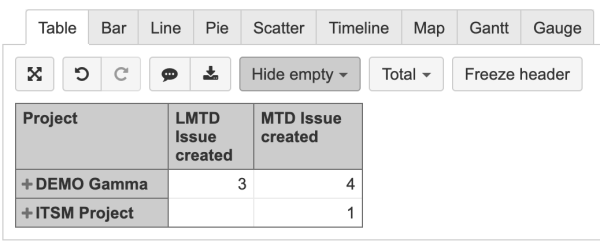# Current Month to Day (MTD) minus Last Month to Day (LMTD)

Hello

I need help to define calculated measure: Current month issues created - last month issues created but with same proportion of days
If today is 25/10 i need issues created from 01/10-25/10 and compare them with 01/09-25/09

## ([Measures].[Issues created], MTD([Time].CurrentMember))

([Measures].[Issues created], MTD([Time].CurrentMember.PrevMember))
something like this

Hi @shaok!
Depending on your use case you can either create a calculated measure that will represent MTD Issues created and LMTD Issues created or you can create calculated member in Time dimension to represent MTD (month to date) and LMTD (last month to parallel date) and then add measures that you are interested in.
I will start by creating calculated member MTD and LMTD in the Time dimension because the same formula will be part of MTD Issues created and LMTD Issue s created calculations below.
The formulas for calculated members in Time dimension:MTD - aggregates dates in the current month till today

``````Aggregate(
PeriodsToDate([Time].[Month],
[Time].[Day].CurrentDateMember)
)
``````LMTD - aggregates dates in a month till day what is Month()-1; meaning the same date what is today, but in the previous month

``````Aggregate(
PeriodsToDate(
[Time].[Month],
[Time].[Day].DateMember(DateSerial(Year(Now()),Month(Now())-1,Day(Now())))
)
)
``````

Example of how it would look with different measures:The formulas for calculated measures in Measure dimension:MTD Issues created

``````Sum(
Filter(
PeriodsToDate([Time].[Month], [Time].[Day].CurrentDateMember),
[Measures].[Issues created]>0
)
)
``````LMTD Issues created

``````Sum(
Filter(
PeriodsToDate([Time].[Month],
[Time].[Day].DateMember(DateSerial(Year(Now()), Month(Now())-1, Day(Now())))
),
[Measures].[Issues created]>0
)
)
``````

Example of how it would look with Project in Rows :